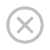# ASP.NET Razor - C# 变量

## 变量

### 实例

```// 使用 var 关键词：
var greeting = "Welcome to W3School";
var counter = 103;
var today = DateTime.Today;

// 使用 data 类型：
string greeting = "Welcome to W3School";
int counter = 103;
DateTime today = DateTime.Today;
```

## 数据类型

int 整数 103, 12, 5168
float 浮点数 3.14, 3.4e38
decimal 小数 1037.196543
bool 逻辑值 true, false
string 字符串值 "Hello W3School", "Bill"

## 运算符

C# 语言支持多种运算符。下面是常见的运算符：

= 为变量赋值。 i=6
• +
• -
• *
• /
• 加上值或变量
• 减去值或变量
• 乘值或变量
• 除值或变量
• i=5+5
• i=5-5
• i=5*5
• i=5/5
• +=
• -=
• 递增变量
• 递减变量
• i += 1
• i -= 1
== 相等。如果值相等，则返回 true。 if (i==10)
!= 不相等。如果值不相等，则返回 true。 if (i!=10)
• <
• >
• <=
• >=
• 小于
• 大于
• 小于等于
• 大于等于
• if (i<10)
• if (i>10)
• if (i<=10)
• if (i>=10)
+ 字符串相加（级联或并置） "w3" + "school"
. 点。分隔对象与方法。 DateTime.Hour
() 括号。对值进行分组。 (i+5)
[] 括号。访问数组或集合中的值。 name
! 非。反转 true 或 false。 if (!ready)
• &&
• ||
• 逻辑与
• 逻辑或

## 转换数据类型

• AsInt()
• IsInt()

{myInt=myString.AsInt();}
• AsFloat()
• IsFloat()

{myFloat=myString.AsFloat();}
• AsDecimal()
• IsDecimal()

{myDec=myString.AsDecimal();}
• AsDateTime()
• IsDateTime()

myDate=myString.AsDateTime();
• AsBool()
• IsBool()

myBool=myString.AsBool();
ToString() 把任意数据类型转换为字符串。 myInt=1234;
myString=myInt.ToString();